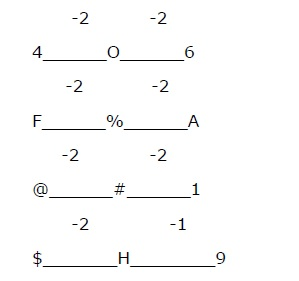# Alpha Numeric Sequence - Solved Examples

Study the following arrangement carefully and answer the question given below −

6 R O T 4 A 8 % B F 1 E # W @ 9 H I \$ M N * 3 2 V \$ 5 G P 7 Q

Q 1 − How many such consonants are there in the above arrangement, each of which is immediately preceded by a consonant and immediately followed by a number?

A - None

B - One

C - Two

D - Three

Explanation

According to question

Consonant –Cosonant-Number

B -F -1

G -P -7

Study the following arrangement carefully and answer the question given below −

6 R 0 T 4 A 8 % B F 1 E # W @ 9 H I \$ M N * 3 2 V \$ 5 G P 7 Q

Q 2 − How many such vowels are there in the above arrangement, each of which is immediately preceded by a letter but immediately not followed by a symbol?

A - None

B - One

C - Two

D - Three

Explanation

According to question

Letter-Vowels-Letter/Number

R - O - T

Study the following arrangement carefully and answer the question given below −

6 R 0 T 4 A 8 % B F 1 E # W @ 9 H I \$ M N * 3 2 V \$ 5 G P 7 Q

Q 3 − Which of the following is the fourth to left of the twelfth from the left end?

A - 8

B - B

C - 2

D - %

Explanation

According to question.

L - L = L

12 - 4 = 8TH

So the required element is %.

Study the following arrangement carefully and answer the question given below −

6 R 0 T 4 A 8 % B F 1 E # W @ 9 H I \$ M N * 3 2 V \$ 5 G P 7 Q

Q 4 − Three of the following four are alike in a certain way based on their positive in the above arrangement and so form a group. Which is the one that does not belong to that group?

A - 406

B - F%A

C - @#1

D - \$H9

Explanation

According to question.Study the following arrangement carefully and answer the question given below −

6 R 0 T 4 A 8 % B F 1 E # W @ 9 H I \$ M N * 3 2 V \$ 5 G P 7 Q

Q 5 − If all the symbols are eliminated from the above arrangement, than which of the following will be the 5th to the right of 10th element from the right end?

A - 4

B - 5

C - V

D - A

Explanation

According to question.

R - R = R

10 - 5 = 5th from right end after deleting all the symbols is 5.

These question based on the following letter /symbol/number sequence. Study it carefully and answer the question.

M 3 R # A P 4 9 @ K D 1 U H 5 \$ 2 N \$ W E Q 6 T V 7 * 8 B X

Q 6 − Which of the following elements is the fourth to the right of 12th from right end?

A - 9

B - 6

C - 5

D - \$

Explanation

Remember, R12 – R4 = R = 6

These question based on the following letter /symbol/number sequence. Study it carefully and answer the question.

M 3 R # A P 4 9 @ K D 1 U H 5 \$ 2 N \$ W E Q 6 T V 7 * 8 B X

Q 7 − How many such numbers are there in the above arrangement which are immediately preceded by a symbol?

A - 2

B - 1

C - None

D - 3

Explanation

The numbers which are preceded by a symbol are \$2,*8.

These question based on the following letter /symbol/number sequence. Study it carefully and answer the question.

M 3 R # A P 4 9 @ K D 1 U H 5 \$ 2 N \$ W E Q 6 T V 7 * 8 B X

Q 8 − If started from M, M is interchanged with 3 and R is interchanged with #and so on than which element will be twelfth from left?

A - 1

B - \$

C - N

D - D

Explanation

As, M 3 R # A P 4 9 @ K D 1

So it will be D

These question based on the following letter /symbol/number sequence. Study it carefully and answer the question.

M 3 R # A P 4 9 @ K D 1 U H 5 \$ 2 N \$ W E Q 6 T V 7 * 8 B X

Q 9 − What will come next in the following sequence-MRP,9KU,52W?

A - QT7

B - 6V8

C - QT*

D - QV7

Explanation

As, M_R_ _P,_9_K_ _U,

_5_2_ _W,_Q_T_ _*.

These question based on the following letter /symbol/number sequence. Study it carefully and answer the question.

M 3 R # A P 4 9 @ K D 1 U H 5 \$ 2 N \$ W E Q 6 T V 7 * 8 B X

Q 10 − Three of the following four are alike in a certain way based on their position in the above arrangement. Which of the following does not belong to the group?

A - M3X

B - R#8

C - P4V

D - KDE

Explanation

M 3 R # A P 4 9 @ K D I U H 5 \$ 2 N \$ W E Q 6 T V 7 * 8 B X

It should be Q in place of E.

reasoning_alpha_numeric_sequence.htm